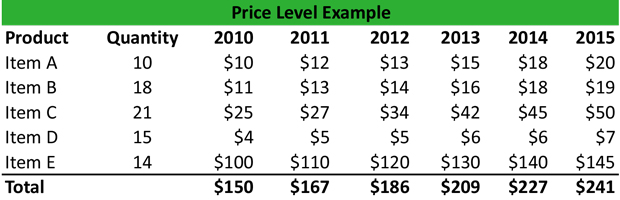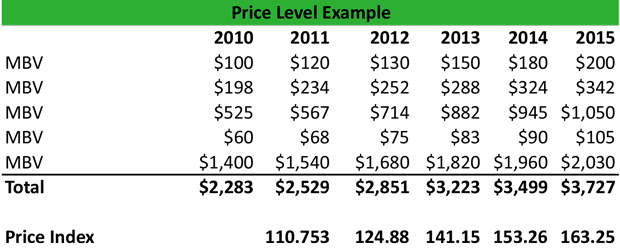# What is a Price Level?

Definition: Price level is the average of all prices of goods and services currently being produced in the economy. This statistic offers an indication of the price of goods and services produced by an economy during a specified period.

## What Does Price Level Mean?

What is the definition of price level? The price level has a significant impact on the purchase of goods and services but also on the purchasing power of money. For instance, if P is the amount of money required to buy a specified quantity of goods and services, then one dollar can buy 1/P.

By considering money as a commodity, its demand will have a negative correlation with its value, and a positive correlation with the price level. The price level changes as the consumer basket of goods and services changes during a specified period, month or year. Furthermore, the price level refers to the price of assets traded on the market.

Let’s look at an example.

## Example

Alex is an economist at the IMF, and he prepares a basket of goods from 2010 to 2015, consisting of five basic items, with the following prices and quantities for the relevant years:Alex wants to calculate the Price Index for each year, but first he has to calculate the market basket values from 2010 to 2015, as follows:

Market Basket 2010 = (10 x \$10) + (18 x \$11) + (21 x \$25) + (15 x \$4) + (15 x \$100) = \$100 + \$198 +\$525 + \$60 +\$1500 = \$2,383

Market Basket 2011 = (10 x \$12) + (18 x \$13) + (21 x \$27) + (15 x \$5) + (14 x \$110) = \$120 + \$234 + \$567 + \$68 + \$1,540 = \$2,529

And so on until he creates the following table:To calculate the price level, Alex takes the value of the market basket 2010 as a base year. Therefore:

2011: MBV 2011 / MBV 2010 x 100 = (\$2,529 / \$2,283) x 100 = 110.75
2012: MBV 2012 / MBV 2010 x 100 = (\$2,851 / \$2,283) x 100 = 124.88
2013: MBV 2013 / MBV 2010 x 100 = (\$3,223 / \$2,283) x 100 = 141.15
2014: MBV 2014 / MBV 2010 x 100 = (\$3,499 / \$2,283) x 100 = 153.26
2015: MBV 2015 / MBV 2010 x 100 = (\$3,727 / \$2,283) x 100 = 163.25

## Summary Definition

Define Price Level: Price level means the average cost of all goods on the market or in a category or the economy.